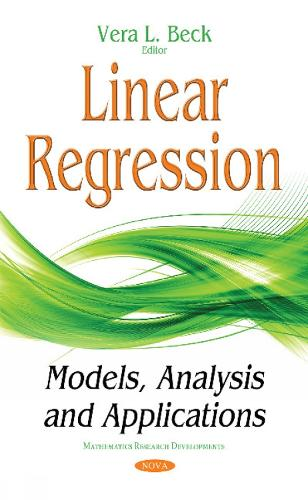•Linear Regression: Models, Analysis & Applications (Hardback)

(editor)
£90.99
Hardback 75 Pages / Published: 01/07/2017
• We can order this

Usually dispatched within 3 weeks

Chapter One addresses the importance of weighted linear regression in fitting straight lines. In Chapter Two, the authors cover the homocedastic condition (ie: variance of ys independent of x, errors of ys accumulative, the heterocedastic case, ie: variance or standard deviation proportional to x values, respectively, and orthogonal regression (error in both axes). The chapter also covers topics such as prediction (using the regression line in reverse), leverage, goodness of fit, comparison between models with and without intercept, uncertainty, polynomial regression models without intercept, and an overview of robust regression through the origin. Chapter Three focuses on linear regression for interval-valued data within the framework of random sets, and proposes a new model that generalises a series of existing ones. Chapter Four provides an investigation on modeling of adsorption of heavy metal ions onto surface-functionalized polymer beads. Linear and non-linear regressions were employed for each of the isotherm models considered to describe the equilibrium data. To reliably assess model validity, various error functions (whose mathematical expressions contain the number of experimental measurements, the numbers of independent variables and parameters in the regression equation as well as the measured and predicted equilibrium adsorption capacities) were used.

Publisher: Nova Science Publishers Inc
ISBN: 9781536119923
Number of pages: 75
Weight: 380 g
Dimensions: 230 x 155 mm